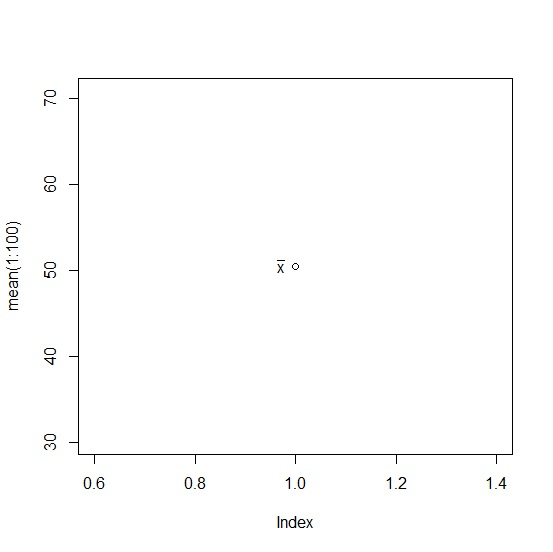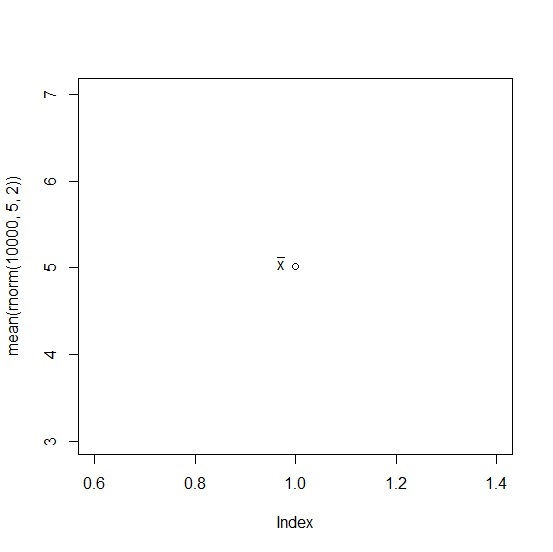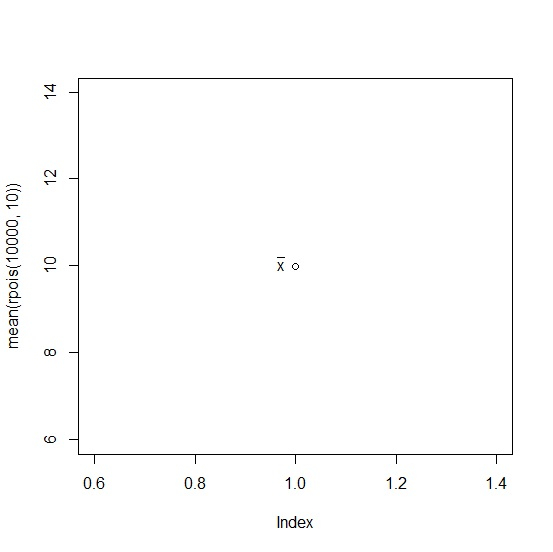# How to display x-bar in base R plot?

To display x-bar in base R plot, we can use text function and define the bar with bar function inside expression function.

For example, if we have a vector called x that contains first ten numbers (1 to 10) then we can display it’s mean inside the base R plot by using the command given below −

text(0.97,5.5,expression(bar("x")))

Check out the examples given below to understand how it works.

## Example 1

Use the code given below to display x-bar in base R plot −

plot(mean(1:100))
text(0.97,50.5,expression(bar("x")))

## Output

If you execute the above given snippet, it generates the following Output −## Example 2

Use the code given below to display x-bar in base R plot −

plot(mean(rnorm(10000,5,2)))
text(0.97,5.05,expression(bar("x")))

## Output

If you execute the above given snippet, it generates the following Output −## Example 3

Use the code given below to display x-bar in base R plot −

plot(mean(rpois(10000,10)))
text(0.97,10.05,expression(bar("x")))

## Output

If you execute the above given snippet, it generates the following Output −